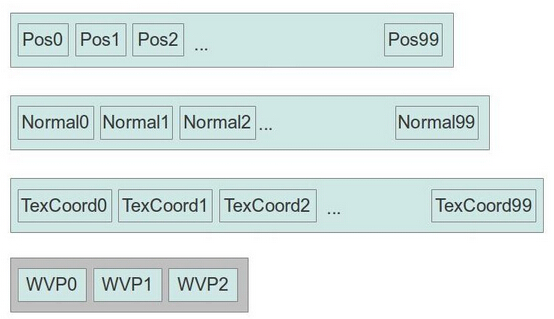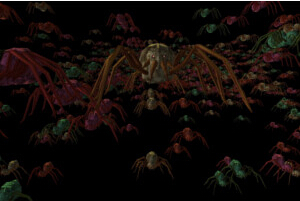modern opengl tutorial

end

# 第三十三课 实例渲染

## 背景## 代码

``````(mesh.h:50)
class Mesh
{
public:
...
void Render(unsigned int NumInstances, const Matrix4f* WVPMats, const Matrix4f* WorldMats);
...
private:
...
#define INDEX_BUFFER 0
#define POS_VB 1
#define NORMAL_VB 2
#define TEXCOORD_VB 3
#define WVP_MAT_VB 4
#define WORLD_MAT_VB 5
GLuint m_VAO;
GLuint m_Buffers;
...``````

``````(mesh.cpp:91)
bool Mesh::InitFromScene(const aiScene* pScene, const string& Filename)
{
...
// Generate and populate the buffers with vertex attributes and the indices
glBindBuffer(GL_ARRAY_BUFFER, m_Buffers[POS_VB]);
glBufferData(GL_ARRAY_BUFFER, sizeof(Positions) * Positions.size(), &Positions,
GL_STATIC_DRAW);
glEnableVertexAttribArray(POSITION_LOCATION);
glVertexAttribPointer(POSITION_LOCATION, 3, GL_FLOAT, GL_FALSE, 0, 0);
glBindBuffer(GL_ARRAY_BUFFER, m_Buffers[TEXCOORD_VB]);
glBufferData(GL_ARRAY_BUFFER, sizeof(TexCoords) * TexCoords.size(), &TexCoords,
GL_STATIC_DRAW);
glEnableVertexAttribArray(TEX_COORD_LOCATION);
glVertexAttribPointer(TEX_COORD_LOCATION, 2, GL_FLOAT, GL_FALSE, 0, 0);
glBindBuffer(GL_ARRAY_BUFFER, m_Buffers[NORMAL_VB]);
glBufferData(GL_ARRAY_BUFFER, sizeof(Normals) * Normals.size(), &Normals,
GL_STATIC_DRAW);
glEnableVertexAttribArray(NORMAL_LOCATION);
glVertexAttribPointer(NORMAL_LOCATION, 3, GL_FLOAT, GL_FALSE, 0, 0);
glBindBuffer(GL_ELEMENT_ARRAY_BUFFER, m_Buffers[INDEX_BUFFER]);
glBufferData(GL_ELEMENT_ARRAY_BUFFER, sizeof(Indices) * Indices.size(), &Indices,
GL_STATIC_DRAW);
glBindBuffer(GL_ARRAY_BUFFER, m_Buffers[WVP_MAT_VB]);
for (unsigned int i = 0; i < 4 ; i++) {
glEnableVertexAttribArray(WVP_LOCATION + i);
glVertexAttribPointer(WVP_LOCATION + i, 4, GL_FLOAT, GL_FALSE, sizeof(Matrix4f),
(const GLvoid*)(sizeof(GLfloat) * i * 4));
glVertexAttribDivisor(WVP_LOCATION + i, 1);
}
glBindBuffer(GL_ARRAY_BUFFER, m_Buffers[WORLD_MAT_VB]);
for (unsigned int i = 0; i < 4 ; i++) {
glEnableVertexAttribArray(WORLD_LOCATION + i);
glVertexAttribPointer(WORLD_LOCATION + i, 4, GL_FLOAT, GL_FALSE, sizeof(Matrix4f),
(const GLvoid*)(sizeof(GLfloat) * i * 4));
glVertexAttribDivisor(WORLD_LOCATION + i, 1);
}
return GLCheckError();
}``````

glVertexAttribDivisor() 函数表面这个属性是实例数据而不是普通的顶点数据，它需要两个参数 —— 第一个参数是属性位置，第二个参数是告诉 OpenGL 在进行实例渲染时实例数据更新的速率，它的基本意义就是在更新实例数据之前会完成多少次顶点数据的渲染。默认情况下 divisor 的值为 0，这种情况下每渲染一个顶点就会对实例数据进行一次更新，如果将这个值设置为 10，这表示前 10 个实例会使用这个缓存中的第一个数据，之后的实例会使用第二个数据，以此类推。因为我们希望的是每个对每个实例使用一个 WVP 矩阵，所有我们将这个值设置为 1。

``````(mesh.cpp:253)
void Mesh::Render(unsigned int NumInstances, const Matrix4f* WVPMats, const Matrix4f* WorldMats)
{
glBindBuffer(GL_ARRAY_BUFFER, m_Buffers[WVP_MAT_VB]);
glBufferData(GL_ARRAY_BUFFER, sizeof(Matrix4f) * NumInstances, WVPMats, GL_DYNAMIC_DRAW);
glBindBuffer(GL_ARRAY_BUFFER, m_Buffers[WORLD_MAT_VB]);
glBufferData(GL_ARRAY_BUFFER, sizeof(Matrix4f) * NumInstances, WorldMats, GL_DYNAMIC_DRAW);
glBindVertexArray(m_VAO);
for (unsigned int i = 0 ; i < m_Entries.size() ; i++) {
const unsigned int MaterialIndex = m_Entries[i].MaterialIndex;
assert(MaterialIndex < m_Textures.size());
if (m_Textures[MaterialIndex]) {
m_Textures[MaterialIndex]->Bind(GL_TEXTURE0);
}
glDrawElementsInstancedBaseVertex(GL_TRIANGLES,
m_Entries[i].NumIndices,
GL_UNSIGNED_INT,
(void*)(sizeof(unsigned int) * m_Entries[i].BaseIndex),
NumInstances,
m_Entries[i].BaseVertex);
}
// Make sure the VAO is not changed from the outside
glBindVertexArray(0);
}``````

for (i = 0 ; i < NumInstances ; i++)
if (i mod divisor == 0)
fetch attribute i/divisor from VBs with instance data
for (j = 0 ; j < NumVertices ; j++)
fetch attribute j from VBs with vertex data

``````(lightning_technique.cpp:25)
#version 330
layout (location = 0) in vec3 Position;
layout (location = 1) in vec2 TexCoord;
layout (location = 2) in vec3 Normal;
layout (location = 3) in mat4 WVP;
layout (location = 7) in mat4 World;
out vec2 TexCoord0;
out vec3 Normal0;
out vec3 WorldPos0;
flat out int InstanceID;
void main()
{
gl_Position = WVP * vec4(Position, 1.0);
TexCoord0 = TexCoord;
Normal0 = World * vec4(Normal, 0.0)).xyz;
WorldPos0 = World * vec4(Position, 1.0)).xyz;
InstanceID = gl_InstanceID;
};``````

VS 中的最后一行代码是进行实例渲染的第二个方法（第一个方法是将实例数据按顶点属性的方式传入）。'gl_InstanceID' 是一个内置变量，它能在 VS 中使用，但是因为我们打算在 FS 中使用它，所以这里我们借助一个输出变量将其传入到 FS 中。gl_InstanceID 是一个整型，所以我们也使用一个整型的输出变量，由于整型数据不能再光栅化阶段进行插值，所以我们需要将这个输出变量标记为 'flat'（如果不这么做会产生编译错误）

``````flat in int InstanceID;
...
uniform vec4 gColor;
...
void main()
{
vec3 Normal = normalize(Normal0);
vec4 TotalLight = CalcDirectionalLight(Normal);
for (int i = 0 ; i < gNumPointLights ; i++) {
TotalLight += CalcPointLight(gPointLights[i], Normal);
}
for (int i = 0 ; i < gNumSpotLights ; i++) {
TotalLight += CalcSpotLight(gSpotLights[i], Normal);
}
FragColor = texture(gColorMap, TexCoord0.xy) * TotalLight * gColor[InstanceID % 4];
};``````

``````(tutorial33.cpp:141)
Pipeline p;
p.SetCamera(m_pGameCamera->GetPos(), m_pGameCamera->GetTarget(), m_pGameCamera->GetUp());
p.SetPerspectiveProj(m_persProjInfo);
p.Rotate(0.0f, 90.0f, 0.0f);
p.Scale(0.005f, 0.005f, 0.005f);
Matrix4f WVPMatrics[NUM_INSTANCES];
Matrix4f WorldMatrices[NUM_INSTANCES];
for (unsigned int i = 0 ; i < NUM_INSTANCES ; i++) {
Vector3f Pos(m_positions[i]);
Pos.y += sinf(m_scale) * m_velocity[i];
p.WorldPos(Pos);
WVPMatrics[i] = p.GetWVPTrans().Transpose();
WorldMatrices[i] = p.GetWorldTrans().Transpose();
}
m_pMesh->Render(NUM_INSTANCES, WVPMatrics, WorldMatrices);``````

mat4 WVP(attribute 3, attribute 4, attribute 5, attribute 6)

## 操作结果